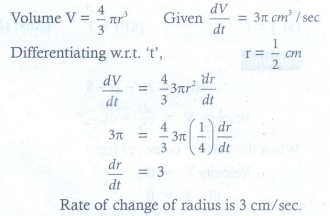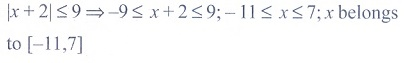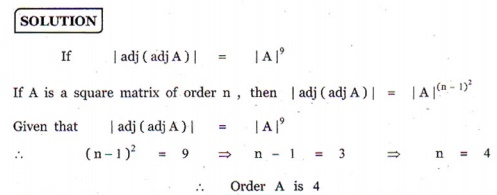# Mathematics - Online Test

Q1. Roots of the algebraic equation x3 + x2 + x + 1 = 0 are
Explaination / Solution:Q2. In a multiple choice question, there are 4 alternatives, of which one or more are correct. The number of ways in which a candidate can attempt this question is
Explaination / Solution:

Since given there are four alternatives in which one or more are correct,we have to consider the following four cases

1 correct answer can be chosen in 4C1 ways = 4 ways

2 correct answer can be chosen in 4C2 ways= 6 ways

3 correct answers can be chosen in  4C3ways  = 4 ways

4 correct answers can be chosen in4C4 ways = 1 way

Hence the totalnumber of ways = 4 + 6 + 4+ 1=15ways

Q3.

The volume of a sphere is increasing in volume at the rate of 3π cm3 / sec .

The rate of change of its radius when radius is 1/2 cm
Explaination / Solution:Q4. If the line 2x – y +  = 0 is a diameter of the circle  then  =
Explaination / Solution:

Equation of circle is

Applying completing the square method

Comparing the above equation with   we get center as (-3,3) and radius as    .

As centre of the circlre lies on diameter , it will satisfy the equation of diameter, so on putting (-3,3) in equation of diameter we get

=>

=>

Q5. If |x + 2| ≤ 9, then x belongs to
Explaination / Solution:Q6. The domain of the function √cosx-1
Explaination / Solution:

This function exists only if cosx−1≥0 ⇒cosx≥1 ⇒cosx>1 OR cosx=1 since maximum value of cosine function is 1 so, cos x >1 is not possible so, cosx=1 cosx=cos2nπ ⇒x=2nπ(n∈I)
Q7. Differential equations are equations containing functions y = f(x), g(x) and
Explaination / Solution:

Differential equations are equations containing functions y = f(x), g(x) and derivatives of y with respect to x.

Q8. If | adj(adj A) |=| A |9 , then the order of the square matrix A is
Explaination / Solution:Q9. Direction cosines of a line are
Explaination / Solution:

a line when intersect another line or axis two kind of angle form ,as an assumption we take positive side of axis, to define the direction of line we take angle made from all three axis.and we take cos not other trigonometric function like sin,tan because we can define Direction cosines of a line are coefficient of i,j,k of a unit vector along that line.

Q10. In a triangle ABC, tan $,$ then tan  is equal to
Explaination / Solution: